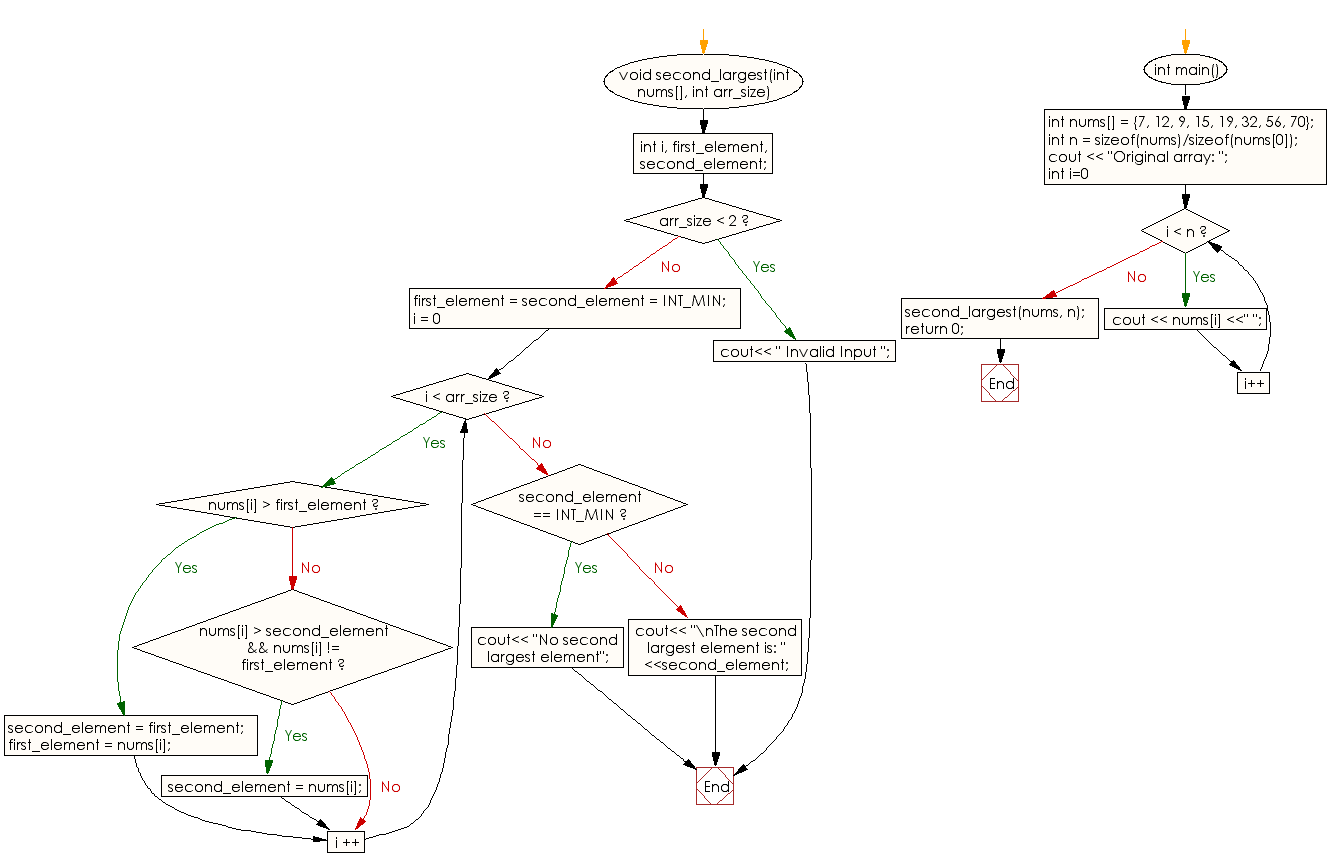﻿ C++ : Find second largest element in an array# C++ Exercises: Find second largest element in a given array of integers

## C++ Array: Exercise-3 with Solution

Write a C++ program to find second largest element in a given array of integers.

Sample Solution:

C++ Code :

``````#include<iostream>
using namespace std;
void second_largest(int nums[], int arr_size)
{
int i, first_element, second_element;

/* There should be atleast two elements */
if (arr_size < 2)
{
cout<< " Invalid Input ";
return;
}

first_element = second_element = INT_MIN;
for (i = 0; i < arr_size ; i ++)
{

if (nums[i] > first_element)
{
second_element = first_element;
first_element = nums[i];
}

else if (nums[i] > second_element && nums[i] != first_element)
{
second_element = nums[i];
}
}
if (second_element == INT_MIN)
{
cout<< "No second largest element";
}
else
{
cout<< "\nThe second largest element is: " <<second_element;
}
}

int main()
{
int nums[] = {7, 12, 9, 15, 19, 32, 56, 70};
int n = sizeof(nums)/sizeof(nums);
cout << "Original array: ";
for (int i=0; i < n; i++)
cout << nums[i] <<" ";
second_largest(nums, n);
return 0;
}
``````

Sample Output:

```Original array: 7 12 9 15 19 32 56 70
The second largest element is: 56
```

Flowchart:C++ Code Editor:

What is the difficulty level of this exercise?

Test your Programming skills with w3resource's quiz.

﻿